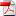# Journal of Applied Mathematics & Bioinformatics

#### Dynamic analysis of Non-Uniform Rayleigh beam Resting on Bi-Parametric Subgrade under Exponentially Varying Moving Loads

•[ Download ]
• Abstract

The response of non-uniform Rayleigh beam resting on bi-parametric subgrades and subjected to exponentially varying magnitude moving load is investigated in this paper. The governing equation is fourth order partial differential equation with variable coefficient. In order to solve this problem, the versatile Galerkin’s method is used to reduce the governing equation to a second order ordinary differential equation. For the solution of this equation, Laplace transformation and convolution theorem are employed. Numerical results in plotted curves are then presented. The results show that response amplitude of the non-uniform Rayleigh beam decreases as the shear modules (G) increases. Also, the deflection profile of the beam decreases with an increasing values of the foundation modulus (k). Furthermore, as the values of the axial force (N), rotatory inertia R0and damping coefficient (e) increases, the response amplitudes of the beam subjected to exponentially varying magnitude moving load decreases. Finally, it was observed that the non-uniform beam undergoes downward deflection profiles from the origin when the effects of each of the parameters such as shear modules, rotatory inertia and damping coefficient on the beam are considered while upward deflection profiles from the origin when the effects of foundation modulus and axial force are noticeable.